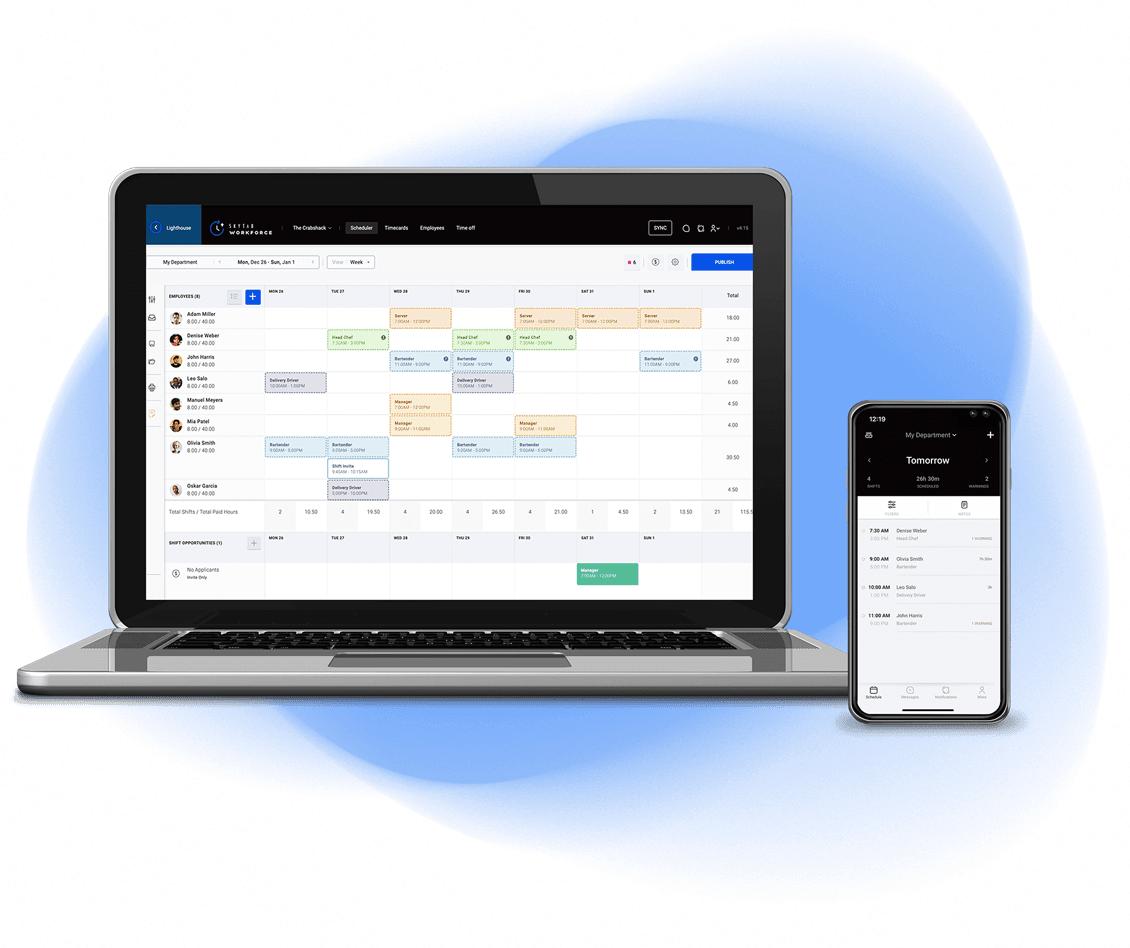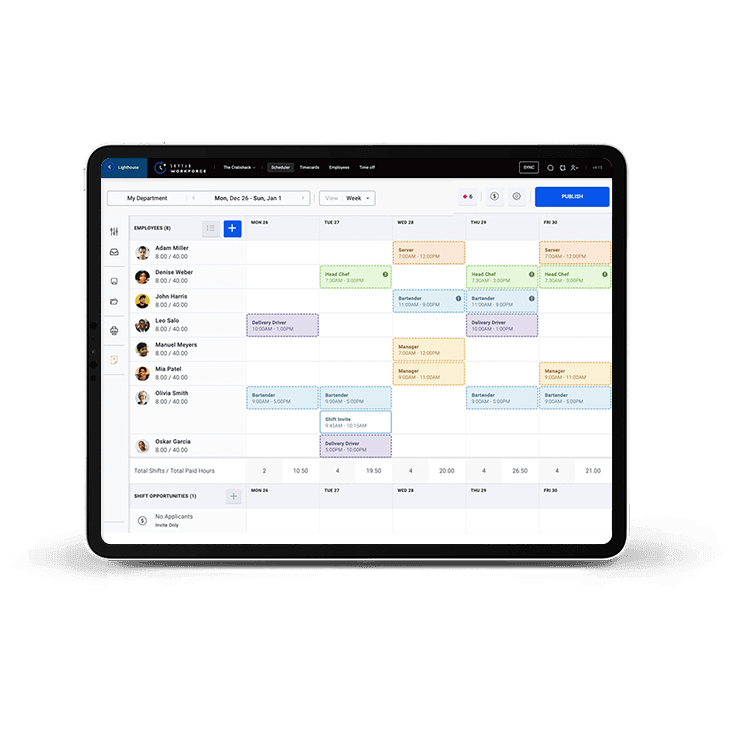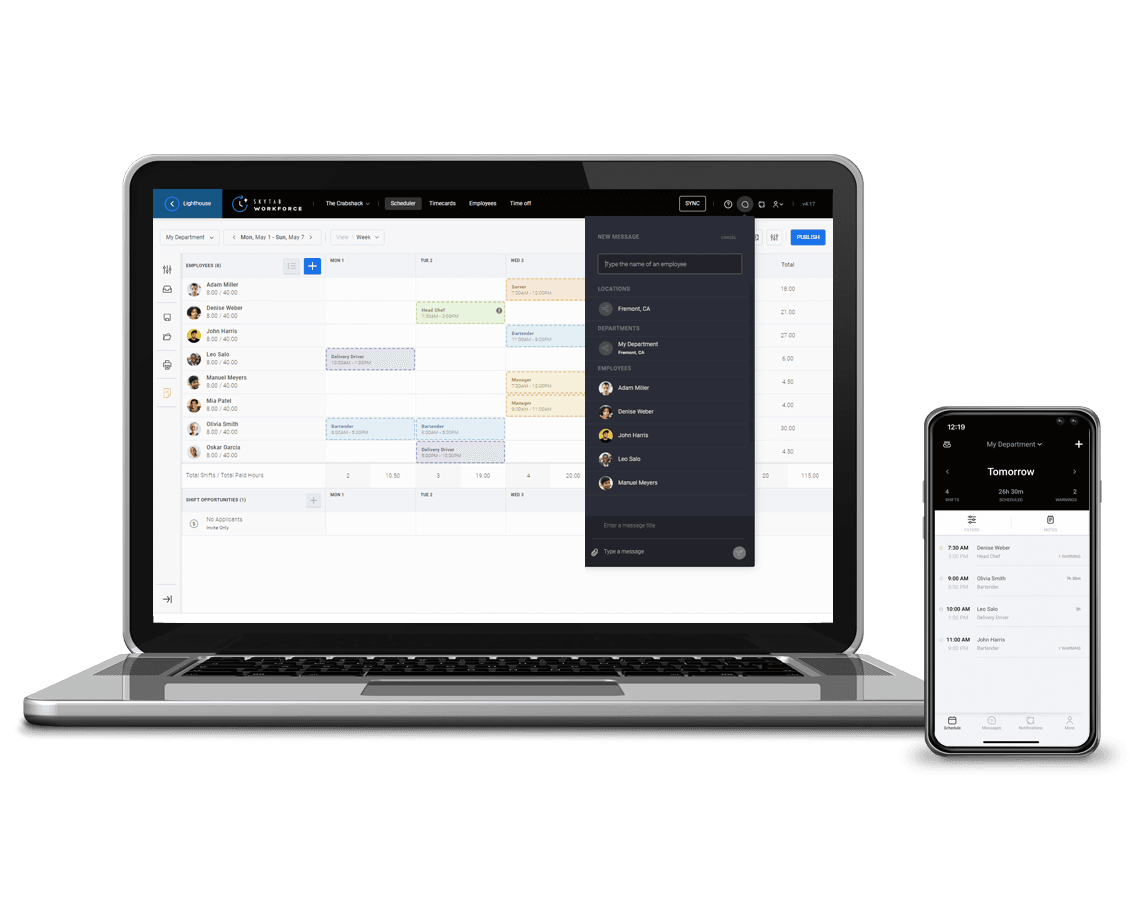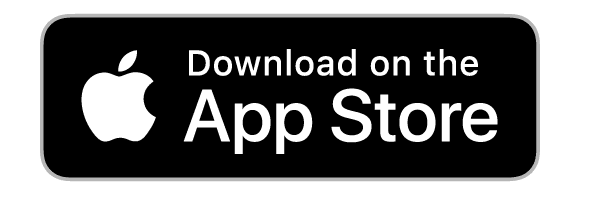# Manage Your Team with Easeself.__wrap_balancer=(t,e,n)=>{n=n||document.querySelector(`[data-br="\${t}"]`);let o=n.parentElement,r=E=>n.style.maxWidth=E+"px";n.style.maxWidth="";let i=o.clientWidth,s=o.clientHeight,c=i/2,u=i,d;if(i){for(;c+1<u;)d=~~((c+u)/2),r(d),o.clientHeight==s?u=d:c=d;r(u*e+i*(1-e))}};self.__wrap_balancer(":R2hlm6:",1)

Make every shift run like clockwork and optimize your workforce with labor management tools.## Build Your Weekly Schedule in Minutesself.__wrap_balancer=(t,e,n)=>{n=n||document.querySelector(`[data-br="\${t}"]`);let o=n.parentElement,r=E=>n.style.maxWidth=E+"px";n.style.maxWidth="";let i=o.clientWidth,s=o.clientHeight,c=i/2,u=i,d;if(i){for(;c+1<u;)d=~~((c+u)/2),r(d),o.clientHeight==s?u=d:c=d;r(u*e+i*(1-e))}};self.__wrap_balancer(":Rb2lm6:",1)

Just a few clicks is all it takes. Build your schedule, communicate changes instantly, and get employee confirmation.

• Easily copy shifts to multiple days of the week
• Create templates to streamline scheduling## Track and Simplify Payrollself.__wrap_balancer=(t,e,n)=>{n=n||document.querySelector(`[data-br="\${t}"]`);let o=n.parentElement,r=E=>n.style.maxWidth=E+"px";n.style.maxWidth="";let i=o.clientWidth,s=o.clientHeight,c=i/2,u=i,d;if(i){for(;c+1<u;)d=~~((c+u)/2),r(d),o.clientHeight==s?u=d:c=d;r(u*e+i*(1-e))}};self.__wrap_balancer(":Rb3lm6:",1)

Streamline accounting to make payroll a breeze. Capture every minute with time card data, control overtime costs, and export payroll.## Stay Compliant with Labor Lawsself.__wrap_balancer=(t,e,n)=>{n=n||document.querySelector(`[data-br="\${t}"]`);let o=n.parentElement,r=E=>n.style.maxWidth=E+"px";n.style.maxWidth="";let i=o.clientWidth,s=o.clientHeight,c=i/2,u=i,d;if(i){for(;c+1<u;)d=~~((c+u)/2),r(d),o.clientHeight==s?u=d:c=d;r(u*e+i*(1-e))}};self.__wrap_balancer(":Rb4lm6:",1)

Curb employee churn. Maintain a fair and healthy work environment while adhering to federal, state, and local labor regulations.## Stay Connected with Real-Time Communicationself.__wrap_balancer=(t,e,n)=>{n=n||document.querySelector(`[data-br="\${t}"]`);let o=n.parentElement,r=E=>n.style.maxWidth=E+"px";n.style.maxWidth="";let i=o.clientWidth,s=o.clientHeight,c=i/2,u=i,d;if(i){for(;c+1<u;)d=~~((c+u)/2),r(d),o.clientHeight==s?u=d:c=d;r(u*e+i*(1-e))}};self.__wrap_balancer(":Rb5lm6:",1)

Keep staff in sync. Accept, swap, and cancel shifts with the Workforce mobile app. Enable push notifications for real-time scheduling information.## Keep a Pulse on Labor from Afarself.__wrap_balancer=(t,e,n)=>{n=n||document.querySelector(`[data-br="\${t}"]`);let o=n.parentElement,r=E=>n.style.maxWidth=E+"px";n.style.maxWidth="";let i=o.clientWidth,s=o.clientHeight,c=i/2,u=i,d;if(i){for(;c+1<u;)d=~~((c+u)/2),r(d),o.clientHeight==s?u=d:c=d;r(u*e+i*(1-e))}};self.__wrap_balancer(":Rb7lm6:",1)

Our InCharge mobile app gives you real-time labor insights on-the-go so you’re always connected with your business.## Want to do More With Back of House Management?self.__wrap_balancer=(t,e,n)=>{n=n||document.querySelector(`[data-br="\${t}"]`);let o=n.parentElement,r=E=>n.style.maxWidth=E+"px";n.style.maxWidth="";let i=o.clientWidth,s=o.clientHeight,c=i/2,u=i,d;if(i){for(;c+1<u;)d=~~((c+u)/2),r(d),o.clientHeight==s?u=d:c=d;r(u*e+i*(1-e))}};self.__wrap_balancer(":Rb8lm6:",1)

SkyTab POS integrates with industry-leading table management, inventory, and many more back of house solutions so you can do more with your restaurant technology.## Find the Best Point of Sale System for Your Businessself.__wrap_balancer=(t,e,n)=>{n=n||document.querySelector(`[data-br="\${t}"]`);let o=n.parentElement,r=E=>n.style.maxWidth=E+"px";n.style.maxWidth="";let i=o.clientWidth,s=o.clientHeight,c=i/2,u=i,d;if(i){for(;c+1<u;)d=~~((c+u)/2),r(d),o.clientHeight==s?u=d:c=d;r(u*e+i*(1-e))}};self.__wrap_balancer(":R1b9lm6:",1)### Full-Service Restaurants### Quick-Service Restaurants### & Bars### Shops### Shops